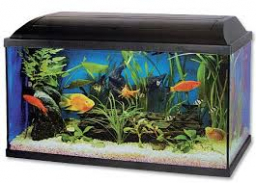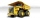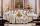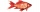# An aquarium

An aquarium tank that measures 2.4 m high, 6 m long, and 1.5 m wide and is completely filled with water. The density of water is 1,000 kg/m3. What is the mass of the water in the tank?

m =  21600 kg

### Step-by-step explanation:Did you find an error or inaccuracy? Feel free to write us. Thank you!Tips to related online calculators
Tip: Our volume units converter will help you with the conversion of volume units.
Tip: Our Density units converter will help you with the conversion of density units.

## Related math problems and questions:

• Air massWhat is the weight of the air in a classroom with dimensions 10 m × 10 m × 2.7 m ? The air density is 1.293 kg/m3.
• MilimetersThe pool is 6 meters long, 3 meters wide and the water in it is filled with water to a height 1.7 m. When John jumped into it and completely submerged, the level has risen by 5.4 mm. How much weight John when we know that one liter of the human body weigh
• Weight of airWhat is the weight of air in the living room measuring width 8 m length 5 m and height 3.1 m? Air density is ρ = 1.2959 kg/m3.The truck with a load capacity of 7 tons has a storage area of 4.5 m and 2.1 m. The weight of 1 m3 of wet sand is 2,000 kg. How high can we load the sand so that the load capacity of the car is not exceeded?
• Iron poleWhat is the mass of a pole with the shape of a regular quadrilateral prism with a length of 1 m and a cross-sectional side length of a = 4.5 cm make from iron with density ρ = 7800 kg/m³?
• Brick wallWhat is the weight of a solid brick wall that is 30 cm wide, 4 m long and 2 m high? The density of the brick is 1500 kg per cubic meter.
• Stones in aquariumIn an aquarium with a length 2 m; width 1.5 m and a depth of 2.5 m is a water level up to three-quarters of the depth. Can we place stones with a volume of 2 m3 into the aquarium without water being poured out?
• HectolitersHow many hectoliters of water fits into cuboid tank with dimensions of a = 3.5 m b = 2.5 m c = 1.4 m?
• Cuboid 5Calculate the mass of the cuboid with dimensions of 12 cm; 0.8 dm and 100 mm made from spruce wood (density = 550 kg/m3).
• The tankThe tank has 1320 liters of water. The tank has the shape of a prism, its base is an rectangle with sides a = 0,6 m and b = 1,5 m. How high does the water level reach in the tank?
• WaterInto a full cylindrical tank high 3 m with a base radius of 2.5 m, we insert cuboid with dimensions 1.7 m, 1.3 m, 1.9 m. How many liters of water will overflow out?
• The glass1 m3 of glass weighs 2600 kg. Calculate the weight of the glass glazing panel with dimensions of 2.5 m and 3.8 m if the thickness of the glass is 0.8 cm
• Glass doorWhat is the weight of glass door panel 5 mm thick height 2.1 meters and a width of 65 cm and 1 cubic dm of glass weighs 2.5 kg?
• AllanAllan keeps tropical fish. His aquarium is 4 feet long, 1 foot wide, and 2 feet tall. Each fish needs at least 0.5ft³ of water. What is the maximum number of fish that he can keep in the aquarium? Please show your solution. Please
• Stones in aquariumIn an aquarium with a length of 2 m, 1.5 m wide, and 2.5 m deep, the water is up to three-quarters of the depth. Can we place 2m cubic meters of stones in the aquarium without spilling water? (0 = no, 1 = yes)
• Water tank 2Water tank cuboid is 12 meters long and 6.5 meters wide and 1.2 meters high. How many hectoliters are in the tank when it is filled to 81%?
• Tess boughtTess bought 6 dressed chickens. Their weights are tagged as follows: 1.4 kg, 1.9 kg, 2.05 kg, 0.94 kg, 1.1 kg and 1.45 kg. What is the total weight of the six chickens